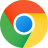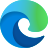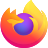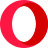# Notes

## Java - swap two numbers without using temp var

author: Paul Kim

categories: java

tags: java

### swap two numbers without using temp var

Note: this code can break due to possibility of overflow, sign handling, not-a-number, and divide-by-zero.

Honestly, I can't think of why anyone would use this in the real world.

public class Main {
public static void main(String[] args) {

// method 1: using + and - operator
int a1 = 123;
int b1 = 456;

a1 = a1 + b1;
b1 = a1 - b1;
a1 = a1 - b1;

System.out.println(a1);
System.out.println(b1);

// method 2: using * and / operator
int a2 = 111;
int b2 = 333;

a2 = a2 + b2;
b2 = a2 - b2;
a2 = a2 - b2;

System.out.println(a2);
System.out.println(b2);

// method 3: using ^ operator
int a3 = 222;
int b3 = 444;

a3 = a3 + b3;
b3 = a3 - b3;
a3 = a3 - b3;

System.out.println(a3);
System.out.println(b3);
}
}© 2021 paulkode.com. All rights reserved.